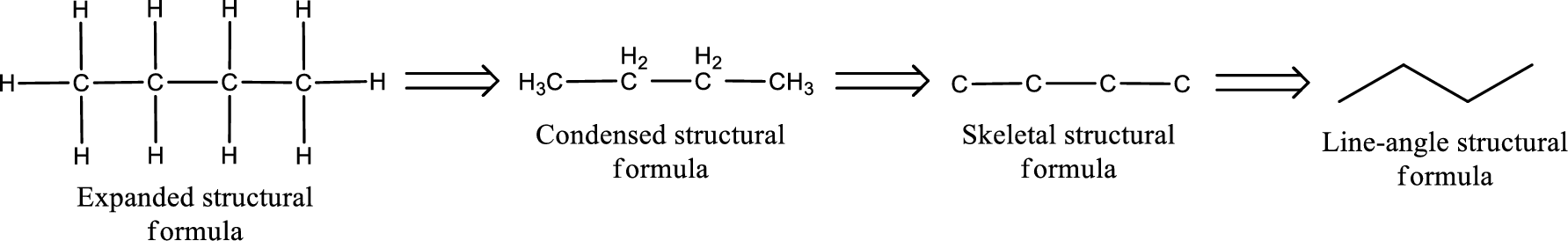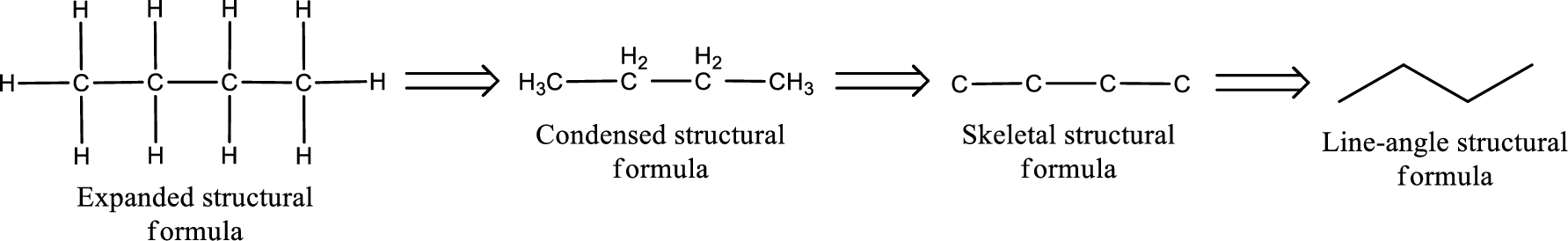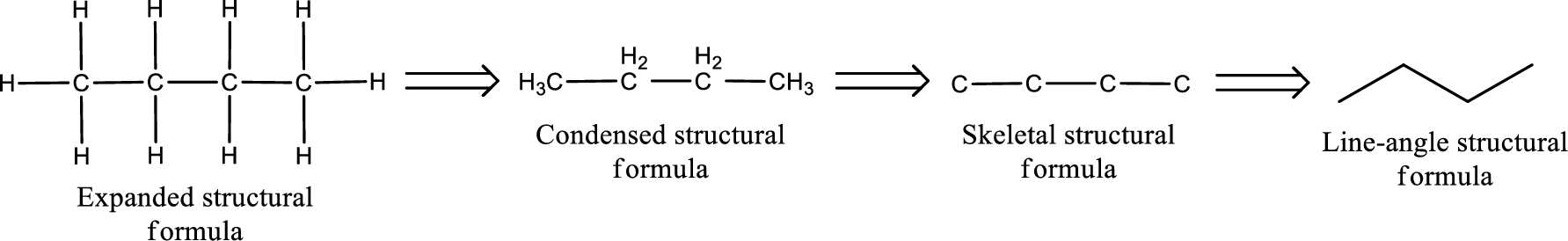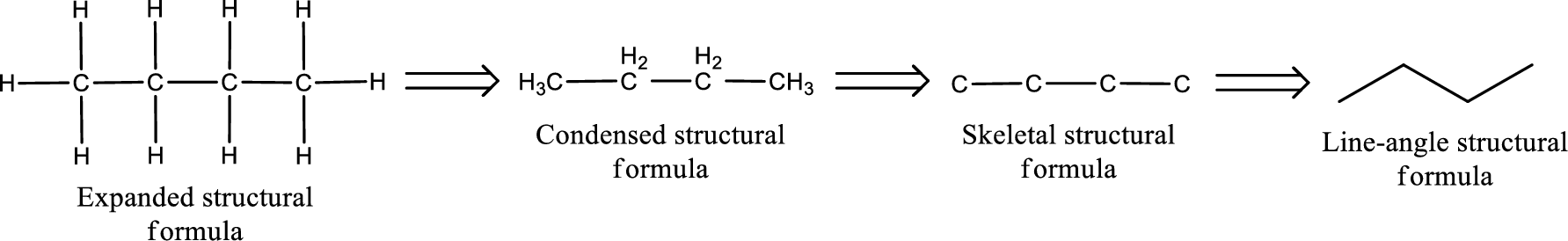Chapter 4, Problem 4.37EP### Organic And Biological Chemistry

7th Edition
STOKER + 1 other
ISBN: 9781305081079

#### Solutions

Chapter
Section### Organic And Biological Chemistry

7th Edition
STOKER + 1 other
ISBN: 9781305081079
Textbook Problem

# Draw a structural formula for each of the following ketones. a. 3-Methyl-2-pentanone b. 3-Hexanone c. Cyclobutanone d. Chloropropanone

(a)

Interpretation Introduction

Interpretation:

Structural formula for the given ketone has to be drawn.

Concept Introduction:

Structure of the ketone can be drawn from the IUPAC name.  In the IUPAC name, the parent chain of carbon atom can be identified and then the substituents present in it can also be identified.  With these information, the structure for the given compound can be drawn.  In a ketone the counting has to be done so that the carbonyl carbon atom gets the least numbering.

The structural representation of organic compound can be done in 2D and 3D.  In two-dimensional representation, there are four types of representation in which an organic compound can be drawn.  They are,

• • Expanded structural formula
• • Condensed structural formula
• • Skeletal structural formula
• • Line-angle structural formula

Structural formula which shows all the atoms in a molecule along with all the bonds that is connecting the atoms present in the molecule is known as Expanded structural formula.

Structural formula in which grouping of atoms are done and in which the central atoms along with the other atoms are connected to them are treated as group is known as Condensed structural formula.

Structural formula that shows the bonding between carbon atoms alone in the molecule ignoring the hydrogen atoms being shown explicitly is known as Skeletal structural formula.

Structural formula where a line represent carbon‑carbon bond and the carbon atom is considered to be present in each point and the end of lines is known as Line-angle structural formula.Explanation

The given name of the compound is 3-methyl-2-pentanone.  From the name it is understood that the parent carbon chain is pentane and it contains five carbon atoms.  The parent chain can be drawn as shown below,

From the name of the given ketone, the substituents that are present can be identified.  In this case, the substituent is a methyl group on third carbon atom.  The carbonyl carbon atom is the second carbon atom...

(b)

Interpretation Introduction

Interpretation:

Structural formula for the given ketone has to be drawn.

Concept Introduction:

Structure of the ketone can be drawn from the IUPAC name.  In the IUPAC name, the parent chain of carbon atom can be identified and then the substituents present in it can also be identified.  With these information, the structure for the given compound can be drawn.  In a ketone the counting has to be done so that the carbonyl carbon atom gets the least numbering.

The structural representation of organic compound can be done in 2D and 3D.  In two-dimensional representation, there are four types of representation in which an organic compound can be drawn.  They are,

• • Expanded structural formula
• • Condensed structural formula
• • Skeletal structural formula
• • Line-angle structural formula

Structural formula which shows all the atoms in a molecule along with all the bonds that is connecting the atoms present in the molecule is known as Expanded structural formula.

Structural formula in which grouping of atoms are done and in which the central atoms along with the other atoms are connected to them are treated as group is known as Condensed structural formula.

Structural formula that shows the bonding between carbon atoms alone in the molecule ignoring the hydrogen atoms being shown explicitly is known as Skeletal structural formula.

Structural formula where a line represent carbon‑carbon bond and the carbon atom is considered to be present in each point and the end of lines is known as Line-angle structural formula.(c)

Interpretation Introduction

Interpretation:

Structural formula for the given ketone has to be drawn.

Concept Introduction:

Structure of the ketone can be drawn from the IUPAC name.  In the IUPAC name, the parent chain of carbon atom can be identified and then the substituents present in it can also be identified.  With these information, the structure for the given compound can be drawn.  In a ketone the counting has to be done so that the carbonyl carbon atom gets the least numbering.

The structural representation of organic compound can be done in 2D and 3D.  In two-dimensional representation, there are four types of representation in which an organic compound can be drawn.  They are,

• • Expanded structural formula
• • Condensed structural formula
• • Skeletal structural formula
• • Line-angle structural formula

Structural formula which shows all the atoms in a molecule along with all the bonds that is connecting the atoms present in the molecule is known as Expanded structural formula.

Structural formula in which grouping of atoms are done and in which the central atoms along with the other atoms are connected to them are treated as group is known as Condensed structural formula.

Structural formula that shows the bonding between carbon atoms alone in the molecule ignoring the hydrogen atoms being shown explicitly is known as Skeletal structural formula.

Structural formula where a line represent carbon‑carbon bond and the carbon atom is considered to be present in each point and the end of lines is known as Line-angle structural formula.(d)

Interpretation Introduction

Interpretation:

Structural formula for the given ketone has to be drawn.

Concept Introduction:

Structure of the ketone can be drawn from the IUPAC name.  In the IUPAC name, the parent chain of carbon atom can be identified and then the substituents present in it can also be identified.  With these information, the structure for the given compound can be drawn.  In a ketone the counting has to be done so that the carbonyl carbon atom gets the least numbering.

The structural representation of organic compound can be done in 2D and 3D.  In two-dimensional representation, there are four types of representation in which an organic compound can be drawn.  They are,

• • Expanded structural formula
• • Condensed structural formula
• • Skeletal structural formula
• • Line-angle structural formula

Structural formula which shows all the atoms in a molecule along with all the bonds that is connecting the atoms present in the molecule is known as Expanded structural formula.

Structural formula in which grouping of atoms are done and in which the central atoms along with the other atoms are connected to them are treated as group is known as Condensed structural formula.

Structural formula that shows the bonding between carbon atoms alone in the molecule ignoring the hydrogen atoms being shown explicitly is known as Skeletal structural formula.

Structural formula where a line represent carbon‑carbon bond and the carbon atom is considered to be present in each point and the end of lines is known as Line-angle structural formula.### Still sussing out bartleby?

Check out a sample textbook solution.

See a sample solution

#### The Solution to Your Study Problems

Bartleby provides explanations to thousands of textbook problems written by our experts, many with advanced degrees!

Get Started